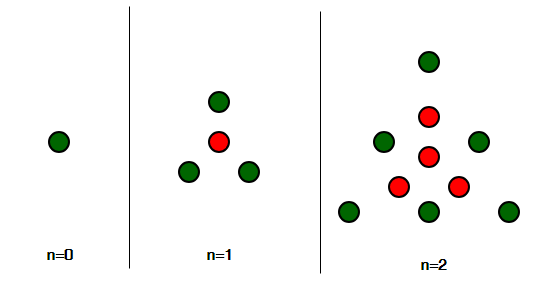Related Articles

# Centered triangular number

• Difficulty Level : Basic
• Last Updated : 03 Aug, 2021

Given an integer n, find the nth Centered triangular number.
Centered Triangular Number is a centered polygonal number that represents a triangle with a dot in the centre and all other dots surrounding the centre in successive triangular layers [Source : Wiki ]

Pictorial Representation :The first few centered triangular number series are :
1, 4, 10, 19, 31, 46, 64, 85, 109, 136, 166, 199, 235, 274, 316, 361, 409, 460………………………..

Examples:

```Input : n = 1
Output : 4
Explanation :
A dot in the centre and 3 dots forming the
triangle outside it, thus 4.

Input : n = 6
Output : 64

Input : n = 10
Output : 166```

Approach
nth Term of centered triangular number is given by:

``

Basic Implementation of the above approach:

## C++

 `// C++ Program to find the``// nth Centered Triangular number``#include ``using` `namespace` `std;` `// function for Centered``// Triangular number``int` `Centered_Triangular_num(``int` `n)``{``    ``// formula for find Centered``    ``// Triangular number nth term``    ``return` `(3 * n * n + 3 * n + 2) / 2;``}` `// Driver Code``int` `main()``{``    ``// For 3rd Centered Triangular number``    ``int` `n = 3;``    ``cout << Centered_Triangular_num(n) << endl;` `    ``// For 12th Centered Triangular number``    ``n = 12;``    ``cout << Centered_Triangular_num(n) << endl;` `    ``return` `0;``}`

## Java

 `// Java Program to find``// the nth Centered``// Triangular number``import` `java.io.*;` `class` `GFG``{``    ` `// function for Centered``// Triangular number``static` `int` `Centered_Triangular_num(``int` `n)``{``    ``// formula for find Centered``    ``// Triangular number nth term``    ``return` `(``3` `* n * n +``            ``3` `* n + ``2``) / ``2``;``}` `// Driver Code``public` `static` `void` `main (String[] args)``{` `// For 3rd Centered``// Triangular number``int` `n = ``3``;``System.out.println(Centered_Triangular_num(n));` `// For 12th Centered``// Triangular number``n = ``12``;``System.out.println(Centered_Triangular_num(n));``}``}` `// This code is contributed by ajit`

## Python3

 `# Program to find nth``# Centered Triangular number` `def` `Centered_Triangular_num(n) :``    ` `    ``# Formula to calculate nth``    ``# Centered Triangular number``    ``return` `(``3` `*` `n ``*` `n ``+``            ``3` `*` `n ``+` `2``) ``/``/` `2` `# Driver Code``if` `__name__ ``=``=` `'__main__'` `:` `    ``# For 3rd Centered``    ``# Triangular number    ``    ``n ``=` `3``    ``print``(Centered_Triangular_num(n))``    ` `    ``# For 12th Centered``    ``# Triangular number``    ``n ``=` `12``    ``print``(Centered_Triangular_num(n))``                ` `                ` `# This code is contributed``# by akt_mit`

## C#

 `// C# Program to find``// the nth Centered``// Triangular number``using` `System;` `class` `GFG``{` `// function for Centered``// Triangular number``static` `int` `Centered_Triangular_num(``int` `n)``{``    ``// formula for find Centered``    ``// Triangular number nth term``    ``return` `(3 * n * n +``            ``3 * n + 2) / 2;``}` `// Driver Code``static` `public` `void` `Main ()``{` `// For 3rd Centered``// Triangular number``int` `n = 3;``Console.WriteLine(Centered_Triangular_num(n));` `// For 12th Centered``// Triangular number``n = 12;``Console.WriteLine(Centered_Triangular_num(n));``}``}` `// This code is contributed by akt_mit`

## PHP

 ``

## Javascript

 ``

Output :

```19
235```

Time Complexity: O(1)
Auxiliary Space: O(1)

Attention reader! Don’t stop learning now. Get hold of all the important DSA concepts with the DSA Self Paced Course at a student-friendly price and become industry ready.  To complete your preparation from learning a language to DS Algo and many more,  please refer Complete Interview Preparation Course.

In case you wish to attend live classes with experts, please refer DSA Live Classes for Working Professionals and Competitive Programming Live for Students.

My Personal Notes arrow_drop_up